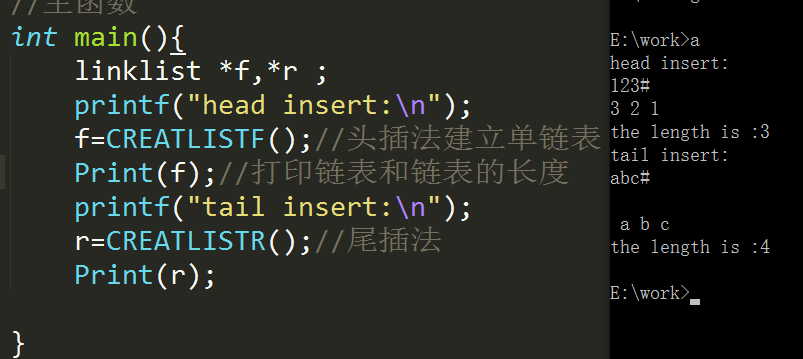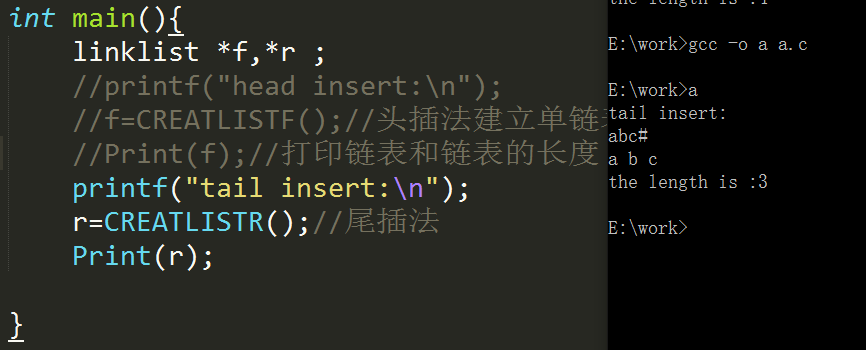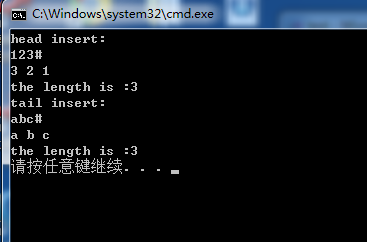`````` #include <stdio.h>
#include <stdlib.h>
#include <malloc.h>

//单链表结构
typedef int datatype;
typedef struct node
{
datatype data;
struct node *next;

/*头插法建表

*/
char ch;//逐个输入字符，以'#'为结束符，返回单链表头指针
ch=getchar();//读入第一个结点的值
while(ch!='#')
{
s->data=ch;
ch=getchar();//读入下一个结点的值
}
}

//计算不带头结点的单链表的长度，然后输出单链表
{
int num=0;
p=L;
while(p)
{
printf("%c ",p->data);
p=p->next;
num++;
}
printf("\nthe length is :%d\n",num);
}
//尾插法——返回表的头指针
char ch;
r=NULL;//尾指针初值为空。
ch=getchar();
while(ch!='#'){
s->data=ch;
else
r->next=s;

r=s;
ch=getchar();
}
if(r!=NULL)
r->next=NULL;
}
//查找——按照序号查找

//主函数
int main(){
//f=CREATLISTF();//头插法建立单链表
//Print(f);//打印链表和链表的长度
printf("tail insert:\n");
r=CREATLISTR();//尾插法
Print(r);

}
``````

=============调用头插法和尾插法，右边是输出结果==========================================只是调用尾插法，右边是输出结果==============================

#4个回答

`````` #include <stdio.h>
#include <stdlib.h>
#include <string.h>
#include <math.h>
//单链表结构
typedef int datatype;
typedef struct node {
datatype data;
struct node *next;

/*头插法建表

*/
char ch; //逐个输入字符，以'#'为结束符，返回单链表头指针
ch = getchar(); //读入第一个结点的值
while (ch != '#') {
s->data = ch;
ch = getchar(); //读入下一个结点的值
}
}

//计算不带头结点的单链表的长度，然后输出单链表
int num = 0;
p = L;
while (p) {
printf("%c ", p->data);
p = p->next;
num++;
}
printf("\nthe length is :%d\n", num);
}
//尾插法——返回表的头指针
char ch;
r = NULL; //尾指针初值为空。
ch = getchar();
while (ch != '#') {
s->data = ch;
else
r->next = s;

r = s;
ch = getchar();
}
if (r != NULL)
r->next = NULL;
}
//查找——按照序号查找

//主函数
int main() {
f=CREATLISTF();//头插法建立单链表
Print(f);//打印链表和链表的长度
fflush(stdin);
printf("tail insert:\n");
r = CREATLISTR(); //尾插法
Print(r);

}
``````（所以第二种，直接调用尾插法是正确的，不存在这个遗留的回车）4 年多之前 回复4 年多之前 回复4 年多之前 回复

#include
#include
#include

//单链表结构
typedef int datatype;
typedef struct node {
datatype data;
struct node *next;

/*头插法建表

*/
char ch; //逐个输入字符，以'#'为结束符，返回单链表头指针
ch = getchar(); //读入第一个结点的值
while (ch != '#') {
s->data = ch;
ch = getchar(); //读入下一个结点的值
}
}

//计算不带头结点的单链表的长度，然后输出单链表
int num = 0;
p = L;
while (p) {
printf("%c ", p->data);
p = p->next;
num++;
}
printf("\nthe length is :%d\n", num);
}
//尾插法——返回表的头指针
char ch;
r = NULL; //尾指针初值为空。
ch = getchar();
while (ch != '#') {
s->data = ch;
else
r->next = s;

``````    r = s;
ch = getchar();
}
if (r != NULL)
r->next = NULL;
``````

}
//查找——按照序号查找

//主函数
int main() {
f=CREATLISTF();//头插法建立单链表
Print(f);//打印链表和链表的长度
printf("tail insert:\n");
r = CREATLISTR(); //尾插法
Print(r);

}

``````
``````